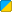# Angle.Forward

(diff) ← Older revision | Latest revision (diff) | Newer revision → (diff)
 Function Syntax Angle:Forward( ) Description: Returns a normalized vector pointing in the direction the angle is pointing Returns: Vector In Object: Angle Realm:BBCode Link: [b][url=http://wiki.garrysmod.com/?title=Angle.Forward]Angle.Forward [img]http://wiki.garrysmod.com/favicon.ico[/img][/url][/b]

## Examples

Description Prints a vector that represents the angle's forward direction to console.```local MyAngle = Angle(0, 0, 90) print("The forward direction of the angle " .. tostring(MyAngle) .. " is " .. tostring(MyAngle:Forward()))``` The forward direction of the angle 0.000 0.000 90.000 is 1.0000 0.0000 -0.0000

• To better understand what forward, right, and up vectors are, straighten out your left arm in such a way that it's perpendicular to your body, pointing to something in front of you. This is a forward vector. Now take your right arm and straighten it out as well, except make this arm point to your right. This is a right vector. Now take either arm and point it upwards, so that it's at a 90 degree angle to your other arm. This is an up vector. Note that the direction that your arms are facing changes as you rotate or tilt your body - it is the same way with Angles.
• An angle describes pitch, yaw, and roll. Pitch and yaw can be thought of as the number of degrees that Vector(1,0,0) must be rotated around the y-axis [ Vector(0,1,0) ] and then the z-axis [ Vector(0,0,1) ] (respectively) to equal this angle's forward vector. An angle's roll is an additional value used for spinning things around their x-axis which does not affect a forward vector at all. However, roll does affect an angle's Right and Up vectors.